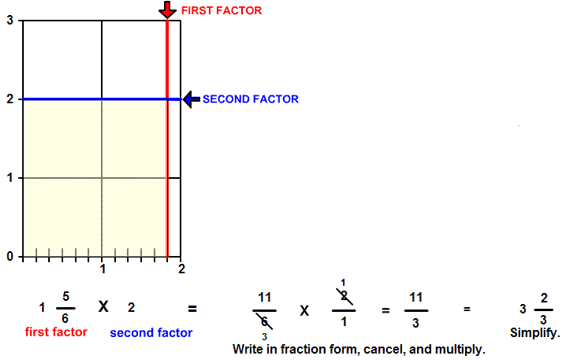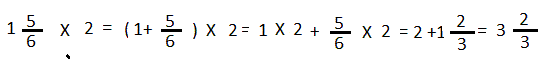# Multiply a mixed number by a whole number

## Multiply a Mixed Number by a Whole Number with Rectangle Models

MULTIPLY MIXED WITH WHOLE
CORRECT:
ATTEMPTS:
SCORE:
PERCENT
WHOLE
NUMERATOR
DENOMINATOR

# MULTIPLY A MIXED NUMBER BY A WHOLE NUMBER INSTRUCTIONS

Follow the directions in the dialog box after pressing the <START> button. The <EXPLAIN> button may be pressed after you enter the second factor to see how to do the example.

The following picture was made by Multiply Fractions with Lines Designer :The parts of a multiplication example are the first factor, the second factor, and the product.

When the program starts, you will be asked to identify the first factor. The first factor is indicated by the red arrow, which shows the horizontal distance along the picture. You will then be asked to identify the second factor. The second factor is indicated by the blue arrow, which shows the vertical distance from 0. The second factor in this program will always be 1, 2, or 3. The program will not continue unless each factor is correctly identified. You will then be asked for the product.

You can see from the picture that the first factor is 1 56 units in length horizontally and the second factor is 2 units in length vertically. The product can be seen as the number of square units within the horizontal and vertical distances.

You can see 2 complete units plus 2 parts. Each part is 56 of a unit. Combine the 3 whole square units and each of the part units for a product of 3 23.

The yellow rectangle gives a picture of the product.

To calculate the product, first write each factor in fraction form as shown in the example. Then multiply the numerators of each factor for the numerator of the product and the denominators of each factor for the denominator of the product.

You may prefer to use a short-cut method known as canceling.To cancel, first write each factor in fraction form. Then think of a number that will divide evenly into any number in the numerator and also any number in the denominator. You can cancel if the number you divide by - the greatest common factor of the numbers - is larger than 1. In the example, 2 will divide into 6 in the denominator of the first factor and into the numerator of the second factor. After you divide by 2, cross off the original term and write the answer near the original term. Then multiply across by the new number instead of the original terms. Canceling will give you smaller numbers to multiply and will give you a product in lowest terms.  Canceling is illustrated below:

How to Cancel will play a short animation that shows you this shortcut way to write a fraction in lowest terms.

Since the second factor in each example of multiply fractions is a whole number, you may use another method to calculate the product as shown in the example below. Multiply the whole number part of 1 56 by the second factor 2 and then the fraction part of 1 56 by the second factor 2. Then add the two numbers for the product.

Written out, the example would look like this:As you can see, you are distributing the factor 2 over the whole number and the fraction part of 1 5/6. When you multiply a factor over two or more addends you are demonstrating the distributive property of multiplication over addition.

For more instruction on multiplying fractions go to How To Multiply Fractions.

After you enter the product you may press the <REPORT> button. The report will ask for your name but you may submit a code for your name. This report will give same results as on the dialog box. The report may be printed or e-mailed.